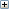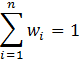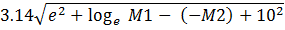﻿ MULT - Multiplier File

# Online Guide to MODFLOW

 Navigation: Groundwater Flow Process MULT - Multiplier File
 Abbreviation in Name file MULT Purpose The Multiplier File  is used to specify multiplier arrays which can be used to calculate layer variables from parameter values. Documentation •Harbaugh, A.W., 2005, MODFLOW-2005, the U.S. Geological Survey modular ground-water model -- the Ground-Water Flow Process: U.S. Geological Survey Techniques and Methods 6-A16. https://doi.org/10.3133/tm6A16 Supported in •MODFLOW-2000 •MODFLOW-2005 •MODFLOW-LGR •MODFLOW-CFP •MODFLOW-NWT •MODFLOW-OWHM Other Notes Multiplier arrays are used in the ADV2, ETS, EVT, HUF2, KDEP, LPF, LVDA, RCH, and UPW input files.

# Input Instructions

Input to define multiplier arrays is read from the file that is specified with "MULT" as the file type. Multiplier arrays can be used to calculate layer variables from parameter values.

FOR EACH SIMULATION

## Data Set 0

[#Text]

Item 0 is optional—“#” must be in column 1. Item 0 can be repeated multiple times.Text

## Data Set 1

NML [MULTPRINT]Explanation of variables

Repeat Item 2 with Items 3 or 4 or 5 until NML multiplier arrays, vectors, or scalars have been defined.

## Data Set 2

MLTNAM [ FUNCTION] [EXPRESSION]MLTNAMFUNCTIONEXPRESSION

## Data Set 3

[RMLT(NCOL,NROW)] - U2DREL

If Item 2 does not contain the optional FUNCTION key word or optional EXPRESSION key word, read item 3. Item 2 cannot have both key words FUNCTION and EXPRESSION at the same time.RMLT

## Data Set 4

[MLTNAM1 [op1 MLTNAM2] [op2 MLTNAM3] [op3 MLTNAM4] ... ] [IPRN]

Item 4 is only read if Item 3 contains the optional FUNCTION key word.Explanation of variables

## Data Set 5 (Only supported in MODFLOW-OWHM)

[Algebraic_Expression]

Item 5 is only read if Item 3 contains the optional EXPRESSION key word. It is a single mathematical expression composed of previously defined multiplier arrays.Algebraic_Expression

## Example Multiplier Array Input Using the FUNCTION and EXPRESSION Keywords in MODFLOW-OWHM.

The following two example inputs illustrate the use of the FUNCTION and EXPRESSION keywords to construct a multiplier array from other multiplier arrays. In the first example, a model layer that has 5 rows and 4 columns has six multiplier arrays, vectors, or scalars to be defined, and, accordingly, the first line of the file contains “6.” The first two arrays (named M1 and M2) are read using the U2DREL utility array reader (Item 3), the third array (named POW) is a multiplier array set to the number 2. The fourth array (named M4) is defined by using the FUNCTION key word, which follows left to right order of operations. This results in calculating M4 as the sum of M1 and M2, which is then raised to power POW (that is, (M1+M2)POW). Note that the "^" operator is only  supported in MODFLOW-OWHM. The fifth matrix (named M5) is defined by using the EXPRESSION key word, which follows mathematical order of operations and produces an identical multiplier to M4. The sixth matrix (named M6) is defined by using the EXPRESSION key word that calculations M2POW first and then sums the result with M1.

The second example is a more complex application of the EXPRESSION key word that constructs a multiplier matrix composed of a weighted power mean,and, of two multiplier matrices (n = 2). In this second example there are eight multiplier arrays, vectors, or scalars to be defined, and accordingly the first line of the file contains “8”. The first two multiplier arrays, vectors, or scalars (named X1 and X2) define x1 and x2. The third multiplier array (named POW) defines the power mean power, p, which for this case is –1 to represent a harmonic mean. The fourth multiplier matrix is a matrix composed of all ones. The fifth multiplier (named W1) is the first weight, w1, read from a free formatted external file. The sixth multiplier (named W2) is the second weight, w2 = 1 – w1, which is constructed using the FUNCTION key word (left to right order of operations). The seventh multiplier (named PowerMean1) solves the power mean using W2 with the EXPRESSION key word. The eighth multiplier (named PowerMean2) solves the power mean without using W2 via the EXPRESSION key word.

## EXAMPLE 1

7

M1

INTERNAL 1.0 (4F6.0) 0

1.0 1.1 1.2 1.3

1.0 1.1 1.2 1.3

2.0 2.2 2.4 2.6

2.0 2.2 2.4 2.6

1.0 1.1 1.2 1.3

M2

INTERNAL 1.0 (4F6.0) 0

5.0 5.1 5.2 5.3

5.0 5.1 5.2 5.3

6.0 6.1 6.2 6.3

6.0 6.1 6.2 6.3

5.0 5.1 5.2 5.3

POW

CONSTANT 2.0

M3 FUNCTION

M1 + M2

M4 FUNCTION

M1 + M2 ^ POW

M5 EXPRESSION

(M1+M2)^POW

M6 EXPRESSION

M1+M2^POW

The resulting values for multiplier M3 are:

6.0 6.2 6.4 6.6

6.0 6.2 6.4 6.6

8.0 8.3 8.6 8.9

8.0 8.3 8.6 8.9

6.0 6.2 6.4 6.6

The resulting values for multipliers M4 and M5 are:

36.00 38.44 40.96 43.56

36.00 38.44 40.96 43.56

64.00 68.89 73.96 79.21

64.00 68.89 73.96 79.21

36.00 38.44 40.96 43.56

The resulting values for multiplier M6 is:

26.00 27.11 28.24 29.39

26.00 27.11 28.24 29.39

38.00 39.41 40.84 42.29

38.00 39.41 40.84 42.29

26.00 27.11 28.24 29.39

## EXAMPLE 2

9

X1                                                   #FIRST NUMBER X1

CONSTANT 25.

X2                                                   #SECOND NUMBER X2

CONSTANT 0.01

POW                                                  #POWER USED IN POWER MEAN, p

CONSTANT -1.0

ONES                                                 #MATRIX OF ALL ONES

CONSTANT 1.0

W1

OPEN/CLOSE FIRST_WEIGHT.TXT 1.0 (FREE) -1

W2 FUNCTION                                          #CALCULATE SECOND WEIGHT AS

ONES - W1

PowerMean1 EXPRESSION                                #POWER MEAN

(W1*X1^POW + W2*X2^POW)^(ONES/POW)

PowerMean2 EXPRESSION                                #POWER MEAN (DOES NOT REQUIRE W2)

(W1*X1^POW + (ONES-W1)*X2^POW)^(ONES/POW)

KeywordEx EXPRESSION                                 #3.14 * SQRT( EXP(POW) + LOG(M1) - NEG(M2) + 10^POW )

Created with Help & Manual 8 and styled with Premium Pack Version 4 © by EC Software